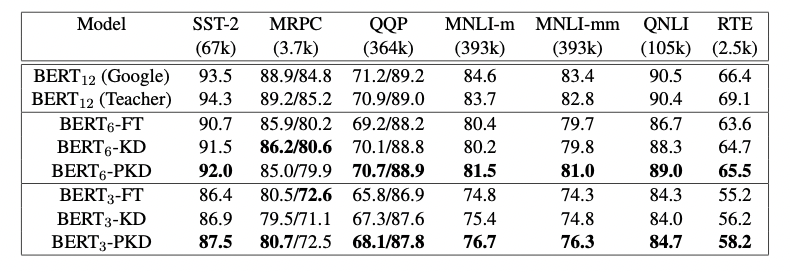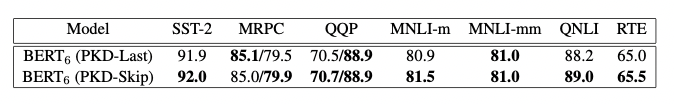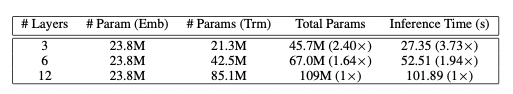# Patient-KD¶

## 1. Patient-KD 简介¶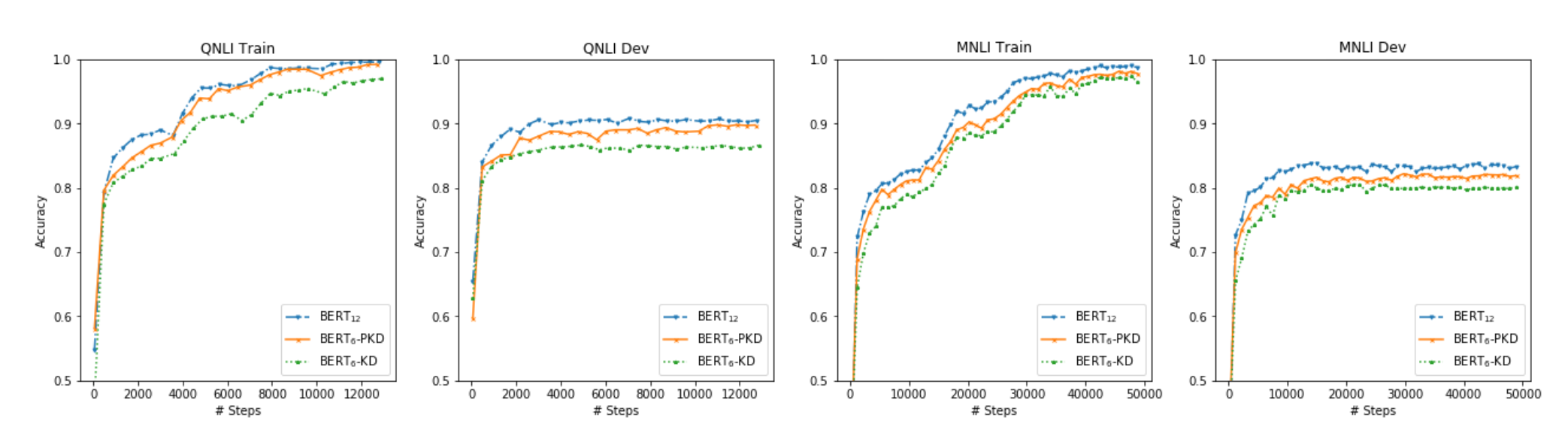BERT预训练模型对资源的高需求导致其很难被应用在实际问题中，为缓解这个问题，论文中提出了Patient Knowledge Distillation（Patient KD）方法，将原始大模型压缩为同等有效的轻量级浅层网络。同时，作者对以往的知识蒸馏方法进行了调研，如图1所示，vanilla KD在QNLI和MNLI的训练集上可以很快的达到和teacher model相媲美的性能，但在测试集上则很快达到饱和。对此，作者提出一种假设，在知识蒸馏的过程中过拟合会导致泛化能力不良。为缓解这个问题，论文中提出一种“耐心”师生机制，即让Patient-KD中的学生模型从教师网络的多个中间层进行知识提取，而不是只从教师网络的最后一层输出中学习。

## 2. 模型实现¶

Patient-KD中提出如下两个知识蒸馏策略：

1. PKD-Skip: 从每k层学习，这种策略是假设网络的底层包含重要信息，需要被学习到（如图2a所示）

2. PKD-last: 从最后k层学习，假设教师网络越靠后的层包含越丰富的知识信息（如图2b所示）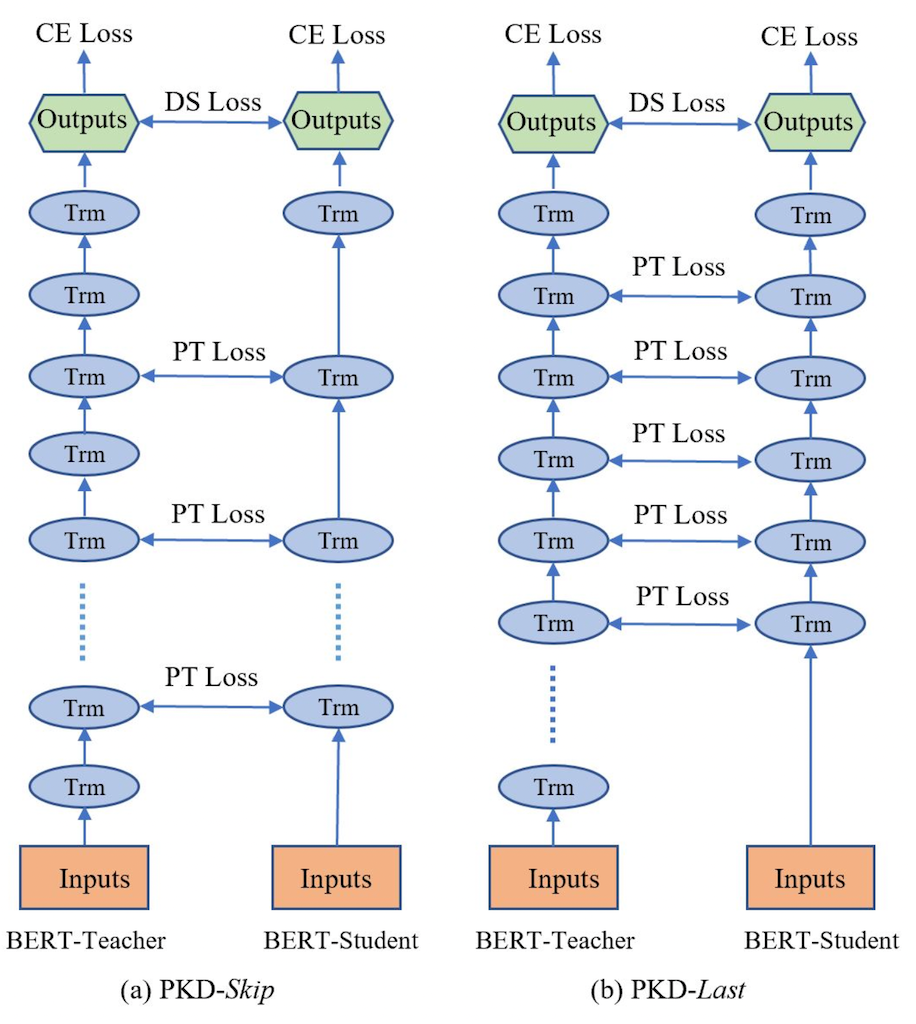$L_{PT} = \sum_{i=1}^{N}\sum_{j=1}^{M} \left \| \frac{h_{i,j}^s}{\left \| h_{i,j}^s \right \|_{2}} - \frac{h_{i, I_{pt}(j)}^t}{\left \| h_{i, I_{pt}(j)}^t \right \|_2}\right \|_2^2$

$h_i = [h_{i,1}, h_{i,2},..., h_{i,k}] = BERT_{k}(x_i) \in \mathbb{R}^{k\times d}​$

$$I_{pt}$$表示要从中提取知识的一组中间层，以从$$BERT_{12}$$压缩到$$BERT_6$$为例，对于PKD-Skip策略，$$I_{pt} = {2,4,6,8,10}$$；对于PKD-Last策略，$$I_{pt} = {7,8,9,10,11}$$。M表示学生网络的层数，N是训练样本的数量，上标s和t分别代表学生网络和教师网络。

$L_{DS}=-\sum_{i \in [N]} \sum_{c \in C}[P^t(y_i = c|x_i;\hat{\theta}^t)\cdot log P^s(y_i = c |x_i; \theta^s)]$
$L_{CE}^s=-\sum_{i \in [N]} \sum_{c \in C}\mathbb{1}[y_i=c]\cdot log P^s(y_i = c|x_i;\theta^s)]$

$L_{PKD} = (1-\alpha)L_{CE}^S+\alpha L_{DS} + \beta L_{PT}$

## 3. 实验结果¶